## 5) Identifying Numbers 6 to 10

Counting: Building Steps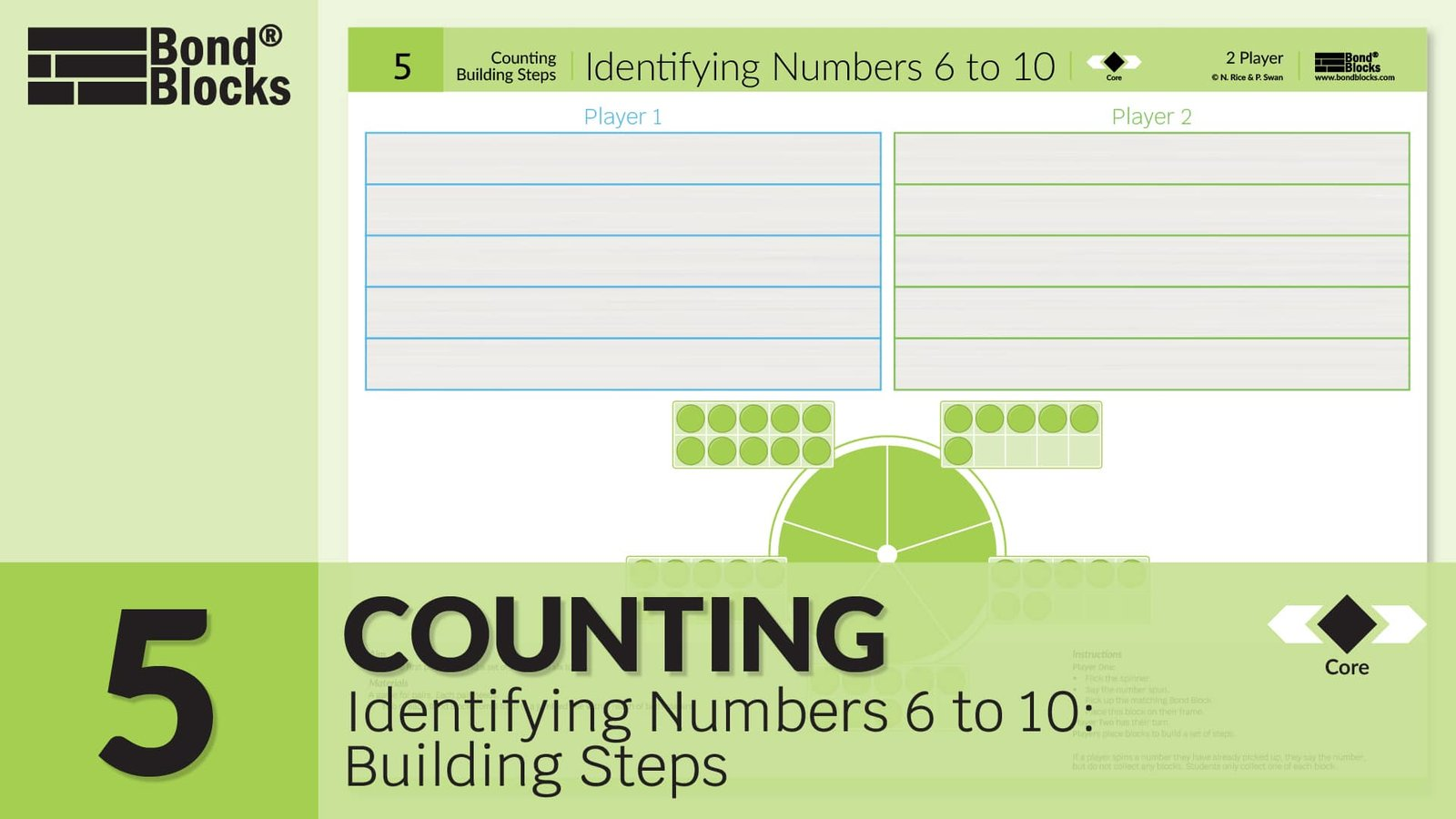### Mathematics

• Develop fluency identifying numbers from 1 to 10 written as numerals.
• Make connections between the written number, said word and quantity of dots.
• Develop fluency identify quantities of dots on ten frames, filled with five-wise filling, from 1 to 10, without counting.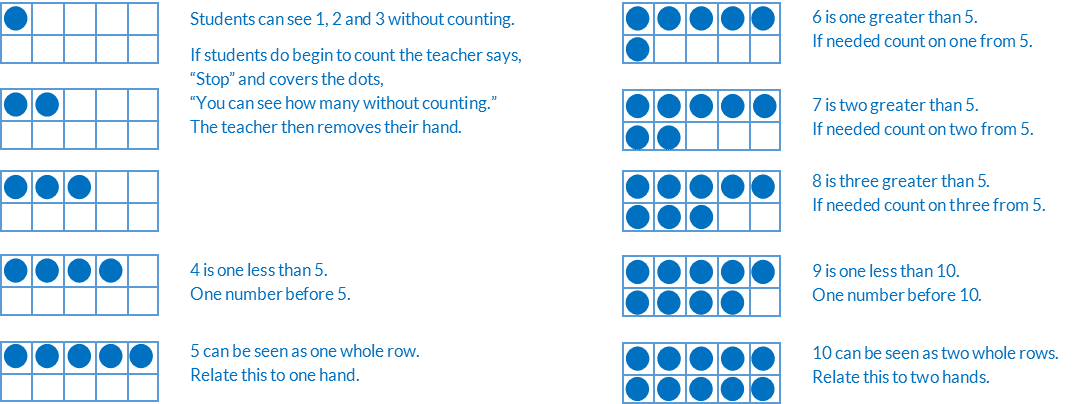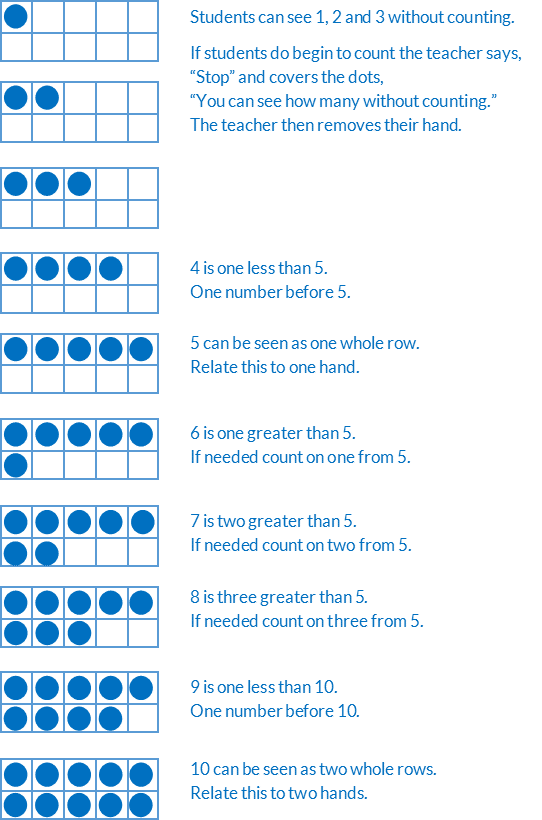It is important that students learn to identify the amount of dots without counting. Counting is only one way to identify how many. Seeing without counting, termed “subitising”, is also essential. Subitising underpins understanding partitioning based addition and subtraction.

Students at mathematical risk over rely on counting by ones to add and subtract. Students should not count on or back, to add or subtract, any more than 2 or at most 3. Any more than this is error prone, ineffective and inhibits the development of appropriate calculation strategies.

• How to structure manipulatives and use fingers to develop addition and subtraction, as opposed to counting .
• Information about students at mathematical risk.

### Language

• one/two/three greater than
• count on one/two/three
• one less than
• one number before

The correct mathematical language when comparing numbers is “greater than” and “less than”. For example, “5 is greater than 3”.

The terms “bigger”, “larger” and “smaller”, in mathematics, refer to size. For example, the 5 block is bigger than the 3 block.

The terms “fewer” and “more” refer to discrete, countable objects. For example, counters.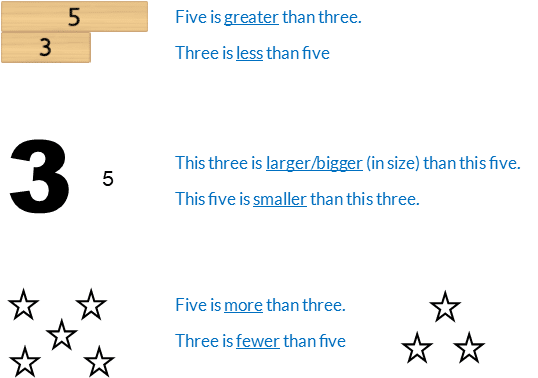## Differentiation

### A little easier

##### Identifying numbers 1 to 5

Use the Activity 5, Counting: Identifying Numbers 1 to 5 Building Steps a little easier game board . This is the same as the core activity but uses numbers 1 to 5.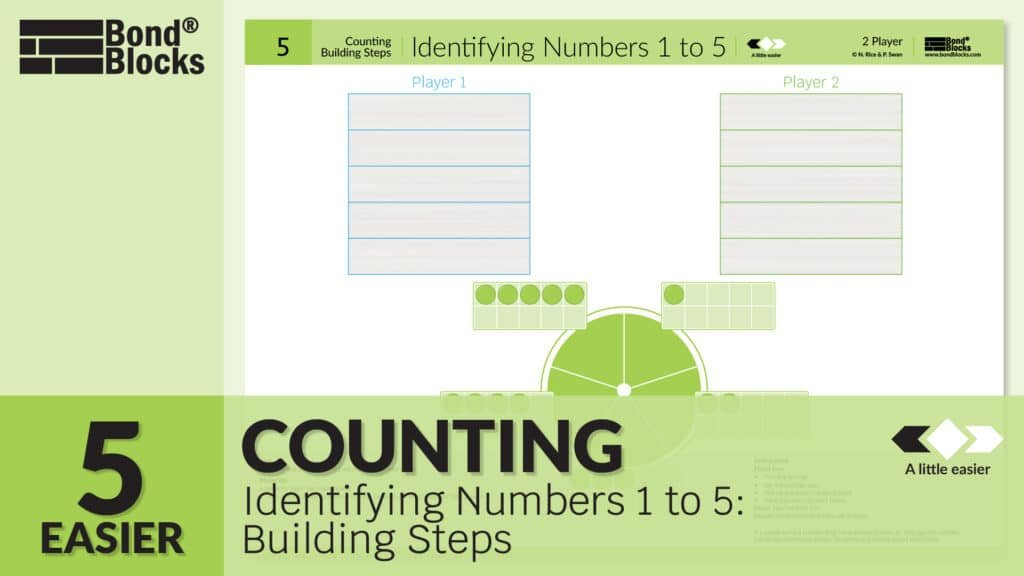##### Consecutive order 6 to 10

Use Activity 5, Counting: Identifying Numbers 6 to 10 Building Steps but do not flick the spinner.

• Instruct the student to move the spinner to six, then collect this block. Repeat for each number in order, moving the spinner clockwise, from six to ten.
• Repeat starting at ten, moving the spinner anticlockwise, from ten to six.

### Progression

In the next chapter of activities students partition the whole of 5 into parts they can see without counting and use these to develop addition and subtraction. Go to

##### Activity 6

Bonds of 5: Bonds, Building a Wall